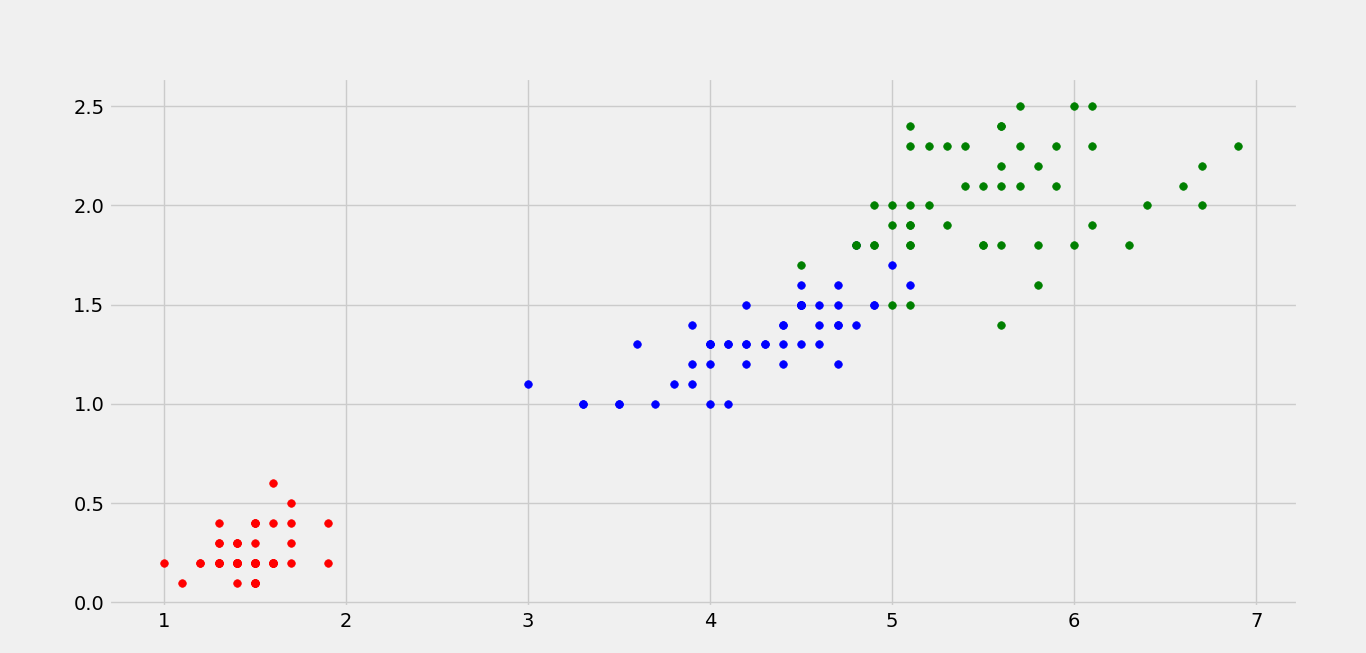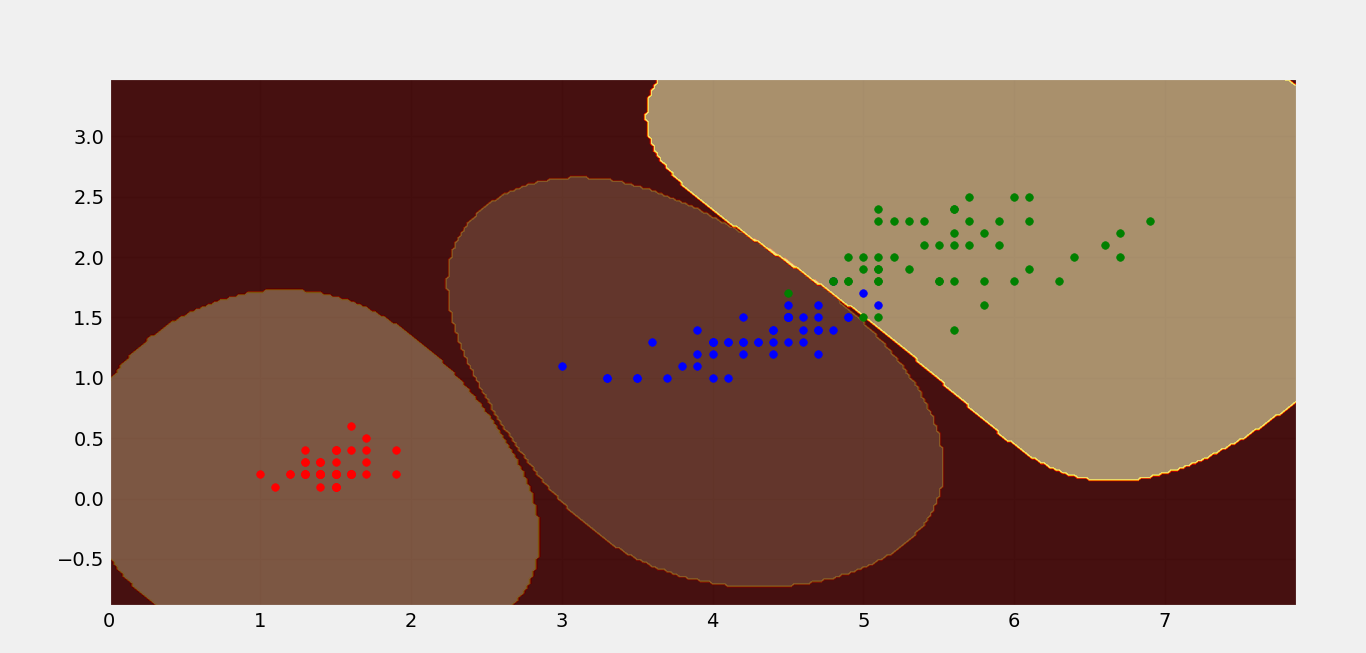# ML | Non-Linear SVM

Prerequisite: Classifying data using SVM

In Linear SVM, the two classes were linearly separable, i.e a single straight line is able to classify both the classes. But imagine if you have three classes, obviously they will not be linearly separable. Therefore, Non-linear SVM’s come handy while handling these kinds of data where classes are not linearly separable.

We will be discussing a Non-Linear Kernel, the RBF kernel, (Radial Basis Function Kernel). So, what this kernel basically does is that it tries to transform the given data into almost linearly separable data.

Let’s consider the example of the IRIS dataset plotted with only 2 of the 4 features (Petal length and Petal Width).

Following is the scatter plot of the same:It’s quite obvious that these classes are not linearly separable. Following is the contour plot of the non-linear SVM which has successfully classified the IRIS dataset using RBF kernel.The above figure shows the classification of the three classes of the IRIS dataset.

1. From sklearn, we imported the SVM library.
2. We created 3 non-linear SVM’s (RBF kernel based).
3. Each SVM was fed with 1 class kept positive and other 2 as negative. Say, SVM1 had labels corresponding to class 1 only else all were made 0. Same for SVM2 and SVM3 respectively.
4. Plot the contour plot of each SVM.
5. Plot the data points.

Below is the Python implementation for the same.

 `import` `numpy as np  ` `import` `pandas as pd  ` `import` `matplotlib.pyplot as plt  ` `from` `matplotlib ``import` `style ` `from` `sklearn.svm ``import` `SVC  ` ` `  `style.use(``'fivethirtyeight'``) ` ` `  `# create mesh grids ` `def` `make_meshgrid(x, y, h ``=``.``02``): ` `    ``x_min, x_max ``=` `x.``min``() ``-` `1``, x.``max``() ``+` `1` `    ``y_min, y_max ``=` `y.``min``() ``-` `1``, y.``max``() ``+` `1` `    ``xx, yy ``=` `np.meshgrid(np.arange(x_min, x_max, h), np.arange(y_min, y_max, h)) ` `    ``return` `xx, yy ` ` `  `# plot the contours ` `def` `plot_contours(ax, clf, xx, yy, ``*``*``params): ` `    ``Z ``=` `clf.predict(np.c_[xx.ravel(), yy.ravel()]) ` `    ``Z ``=` `Z.reshape(xx.shape) ` `    ``out ``=` `ax.contourf(xx, yy, Z, ``*``*``params) ` `    ``return` `out ` ` `  `color ``=` `[``'r'``, ``'b'``, ``'g'``, ``'k'``] ` ` `  `iris ``=` `pd.read_csv(``"iris-data.txt"``).values ` ` `  ` `  `features ``=` `iris[``0``:``150``, ``2``:``4``] ` `level1 ``=` `np.zeros(``150``) ` `level2 ``=` `np.zeros(``150``) ` `level3 ``=` `np.zeros(``150``) ` ` `  `# level1 contains 1 for class1 and 0 for all others. ` `# level2 contains 1 for class2 and 0 for all others. ` `# level3 contains 1 for class3 and 0 for all others. ` `for` `i ``in` `range``(``150``): ` `    ``if` `i>``=` `0` `and` `i<``50``: ` `        ``level1[i] ``=` `1` `    ``elif` `i>``=` `50` `and` `i<``100``: ` `        ``level2[i] ``=` `1` `    ``elif` `i>``=` `100` `and` `i<``150``: ` `        ``level3[i]``=` `1` ` `  `# create 3 svm with rbf kernels ` `svm1 ``=` `SVC(kernel ``=``'rbf'``) ` `svm2 ``=` `SVC(kernel ``=``'rbf'``) ` `svm3 ``=` `SVC(kernel ``=``'rbf'``) ` ` `  `# fit each svm's ` `svm1.fit(features, level1) ` `svm2.fit(features, level2) ` `svm3.fit(features, level3) ` ` `  `fig, ax ``=` `plt.subplots() ` `X0, X1 ``=` `iris[:, ``2``], iris[:, ``3``] ` `xx, yy ``=` `make_meshgrid(X0, X1) ` ` `  `# plot the contours ` `plot_contours(ax, svm1, xx, yy, cmap ``=` `plt.get_cmap(``'hot'``), alpha ``=` `0.8``) ` `plot_contours(ax, svm2, xx, yy, cmap ``=` `plt.get_cmap(``'hot'``), alpha ``=` `0.3``) ` `plot_contours(ax, svm3, xx, yy, cmap ``=` `plt.get_cmap(``'hot'``), alpha ``=` `0.5``) ` ` `  `color ``=` `[``'r'``, ``'b'``, ``'g'``, ``'k'``] ` ` `  `for` `i ``in` `range``(``len``(iris)): ` `    ``plt.scatter(iris[i][``2``], iris[i][``3``], s ``=` `30``, c ``=` `color[``int``(iris[i][``4``])]) ` `plt.show() `

My Personal Notes arrow_drop_upCheck out this Author's contributed articles.

If you like GeeksforGeeks and would like to contribute, you can also write an article using contribute.geeksforgeeks.org or mail your article to contribute@geeksforgeeks.org. See your article appearing on the GeeksforGeeks main page and help other Geeks.

Please Improve this article if you find anything incorrect by clicking on the "Improve Article" button below.

Article Tags :
Practice Tags :

Be the First to upvote.

Please write to us at contribute@geeksforgeeks.org to report any issue with the above content.